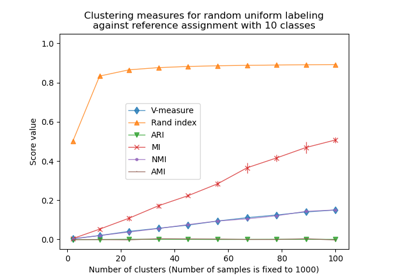# sklearn.metrics.normalized_mutual_info_score¶

sklearn.metrics.normalized_mutual_info_score(labels_true, labels_pred, *, average_method='arithmetic')[source]

Normalized Mutual Information between two clusterings.

Normalized Mutual Information (NMI) is a normalization of the Mutual Information (MI) score to scale the results between 0 (no mutual information) and 1 (perfect correlation). In this function, mutual information is normalized by some generalized mean of H(labels_true) and H(labels_pred)), defined by the average_method.

This measure is not adjusted for chance. Therefore adjusted_mutual_info_score might be preferred.

This metric is independent of the absolute values of the labels: a permutation of the class or cluster label values won’t change the score value in any way.

This metric is furthermore symmetric: switching label_true with label_pred will return the same score value. This can be useful to measure the agreement of two independent label assignments strategies on the same dataset when the real ground truth is not known.

Read more in the User Guide.

Parameters:
labels_trueint array-like of shape (n_samples,)

A clustering of the data into disjoint subsets.

labels_predint array-like of shape (n_samples,)

A clustering of the data into disjoint subsets.

average_method{‘min’, ‘geometric’, ‘arithmetic’, ‘max’}, default=’arithmetic’

How to compute the normalizer in the denominator.

New in version 0.20.

Changed in version 0.22: The default value of average_method changed from ‘geometric’ to ‘arithmetic’.

Returns:
nmifloat

Score between 0.0 and 1.0 in normalized nats (based on the natural logarithm). 1.0 stands for perfectly complete labeling.

v_measure_score

V-Measure (NMI with arithmetic mean option).

adjusted_rand_score

adjusted_mutual_info_score

Examples

Perfect labelings are both homogeneous and complete, hence have score 1.0:

>>> from sklearn.metrics.cluster import normalized_mutual_info_score
>>> normalized_mutual_info_score([0, 0, 1, 1], [0, 0, 1, 1])
...
1.0
>>> normalized_mutual_info_score([0, 0, 1, 1], [1, 1, 0, 0])
...
1.0


If classes members are completely split across different clusters, the assignment is totally in-complete, hence the NMI is null:

>>> normalized_mutual_info_score([0, 0, 0, 0], [0, 1, 2, 3])
...
0.0


## Examples using sklearn.metrics.normalized_mutual_info_score¶Adjustment for chance in clustering performance evaluation

Adjustment for chance in clustering performance evaluation